# Calculator for Cross Section, Mass, Axial & Polar Area Moment of Inertia and Section Modulus

This online calculator computes the axial and polar area moments of inertia (also known as second moment of area or second area moment), the section modulus, the outer-fibre distance and the cross sectional area of many beams. From many surfaces, the torsional moment of inertia and the torsionial section modulus can also be calculated.

In addition, the mass can be computed, too. Steel, aluminum and different types of wood are available as material. At the bottom of the page, the formulas for the axial area moment of inertia and section modulus are listed in a table.

## Calculator for Area Moment of Inertia and Section Modulus

By default, one can calculate the moments, mass and cross section for an I-beam (I100).

 cross section circle with slot with hole pipe semi-circle rectangle rect. with hole rect. + hole rectangle-pipe with hole I/H-section I/H with hole U/C-section U/C with hole T-section L-section L rotated triangle hexagon octagon height H mm width B mm material * steel aluminium spruce pine oak beech
 diameter d mm height h mm width b mm length of beam * m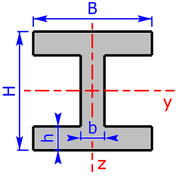units:  mm     cm
can be changed later, too

 2nd area moment section modulus in mm4 in mm3 Iy Wy Iz Wz It Wt Ip
 cross section mm2 mass kg

outer-fibre distance:

* You have to fill in these fields only if the mass should be calculated, too. Only the smallest section modulus will be calculated!

### Explanation of the abbreviations

 Dm diameter in mm Iy, Iz axial area moments of inertia Wy, Wz section modulus It torsional moment of inertia Wt torsional section modulus Ip polar area moments of inertia: Ip = Iy + Iz for circular cross sections: It = Ip e1-4 outer-fibre distance, see following section

### Outer-fibre distance

The outer-fibre distance is the distance from the neutral fiber to the outer fibre. For homo­geneous cross-sections, the neutral fibre always runs through the center of gravity SP of the surface which lies in the center of the coordinate system. The 4 outer fibres are the furthest away from the respective coordinate axes.

The sketch shows which lengths denote the four outer-fiber distances e1, e2, e3 and e4.

Therefore the lengths e1 and e2 are the vertical outer-fibre distances and the lengths e3 and e4 are to the horizontal outer-fibre distances.

## Manual

• The following cross sectional areas are available, whereby profiles marked by * can have a clearance hole (bore), too:
• circle, with slot too *
• pipe / hollow circular
• semi-circle
• rectangle-section *
• rectangle-pipe / hollow rectangular *
• I/H-section (I/H-beam) *
• U/C-section (U/C-beam) *
• T-section (T-beam)
• L-section (angle section), isosceles and not isosceles
• L-section (isosceles) rotated through 45°
• isosceles triangle
• hexagon / six-sided figure
• octagon / eight-sided figure

• Below you will find sketches of all cross sectional areas. The cross sectional areas must always be symmetrical to the two coordinate axes.
• All white fields have to be completed. Results are displayed on a green background.
• Accuracy can not be guaranteed - for corrections or additions please use my contact form!

## Background Knowledge and Formulas

In the following table you will find the formulas for the axial area moments of inertia and the section modulus. Then the mathematical relationship between these two quantities is explained.

### Formulas for axial Area Moments of Inertia & Section Modulus

The following relationships apply to all the formulas listed in the table below:

• b3 = B - b
• b4 = B - 2·b
• h3 = H - h
• h4 = H - 2·h

 Cross Section Axial Area Moment of Inertia Section Modulus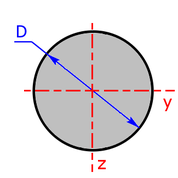circle-section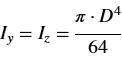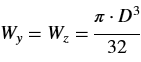pipe-sectionrectangle-section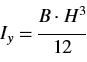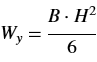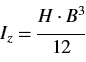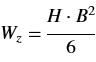rectangle-pipeb4 = B - 2·b          h4 = H - 2·h         (b4 and h4 = inside dimensions)I/H-sectionb3 = B - b          h4 = H - 2·hC/U-section b3 = B - b h4 = H - 2·h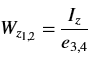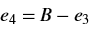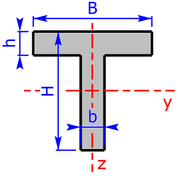T-section b3 = B - b h3 = H - h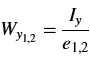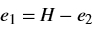L-section b3 = B - b h3 = H - h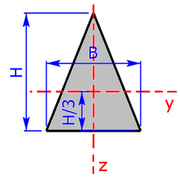triangle-section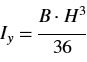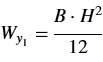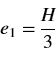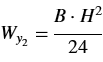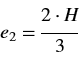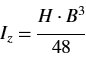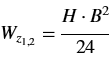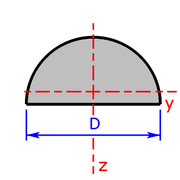semi-circle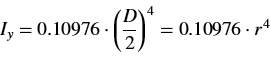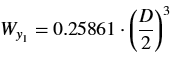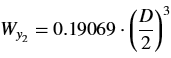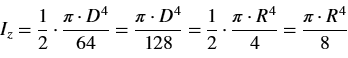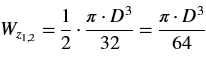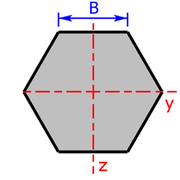hexagon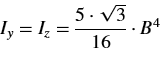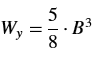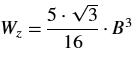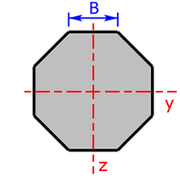octagon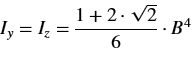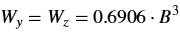### Correlation Section Modulus < > Area Moment of Inertia

The section modulus can be calculated by the following formulas if the area moment of inertia and the outer-fibre distance are known.

The section modulus Wy relative to the y-axis is:

The section modulus Wz relative to the z-axis is:

 Iy area moment of inertia relative to the y-axis Iz area moment of inertia relative to the z-axis e1 lower outer-fibre distance in the z-direction e2 upper outer-fibre distance in the z-direction e3 left outer-fibre distance in the y-direction e4 right outer-fibre distance in the y-direction

If the cross sectional area is not symmetrical to an axis (e1 ≠ e2 and/or e3 ≠ e4), there are two different section modulus relative to this axis, see figure above. Only the smallest section modulus will be calculated!

### Comparison: idealized model and real I-beam I100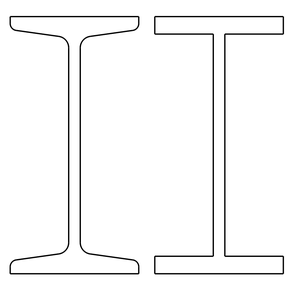Figure 1: On the left a narrow I-beam I100, on the right the simplified model.

In figure 1 you can see a narrow I-beam I100 on the left, on the right you find a simplified model, as it used by the calculator.

The variations in the calculation arise from the fact that the real I-beam has oblique flange surfaces and the inner edges are rounded. This can be seen very well in figure 1.

All drawings were created by using the free programs FreeCAD and GIMP.

The following table compares the calculated values and the real values:

 Iy in cm4 Wy in cm3 Iz in cm4 Wz in cm3 A in mm2 m' in kg/m calculated values 172.1 34.4 14.2 5.7 1069 8.40 real values 171 34.2 12.2 4.88 1060 8.34 variations in % 0.64 0.58 16.4 16.8 0.85 0.72

As you can see, Iy and Wy, the cross sectional area A and the mass per meter match very well. The z-values differ slightly more, but are still useful as an estimate.

### Sketches of the available cross sectional areas

These 20 profiles can be selected as a cross sectional area on the calculator: